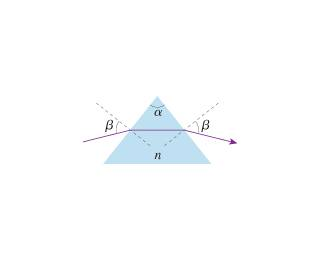# Law of Refraction question

## Homework Statement

There's one angle of incidence β onto a prism for which the light inside an isosceles prism travels parallel to the base and emerges at angle β.
(Figure 1)

## Homework Equations

Find an expression for β in terms of the prism's apex angle α and index of refraction n.
http://session.masteringphysics.com/problemAsset/1384202/2/23.P58.jpg

## The Attempt at a Solution

to Use refraction law: n1sin(betta1)=n2sin(betta2). Then express betta2 through alpha. But i am not getting the right answer

ehild
Homework Helper

## Homework Statement

There's one angle of incidence β onto a prism for which the light inside an isosceles prism travels parallel to the base and emerges at angle β.
(Figure 1)

## Homework Equations

Find an expression for β in terms of the prism's apex angle α and index of refraction n.## The Attempt at a Solution

to Use refraction law: n1sin(betta1)=n2sin(betta2). Then express betta2 through alpha. But i am not getting the right answer